GEATbx: Main page  Tutorial  Algorithms  M-functions  Parameter/Options  Example functions  www.geatbx.com

# 7 Multi-objective Optimization

In many real world problems there are several criteria which have to be considered in order to evaluate the quality of an individual. Only on the basis of the comparison of these several criteria or objectives (thus multi-objective) can a decision be made as to the superiority of one individual over another. Then, as in single-objective problems, an order of individuals within the population can be established from these reciprocal comparisons - multi-objective ranking. After this order has been established the single-objective ranking methods from the subsection 3.1 can be used to convert the order of the individuals to corresponding fitness values.

Multi-objective fitness assignment (and with it multi-objective optimization ) is concerned with the simultaneous minimization of NObj criteria fr, with r = 1, ..., NObj. The values fr are determined by the objective function, which in turn is dependent on the variables of the individuals (the decision variables).

A straightforward example should serve as the motivation for the following considerations. When objects are produced, the production costs should be kept low and the objects should be produced quickly. Various solutions can be developed during production planning which may differ regarding the number and type of the machines employed, as well as regarding the number of workers. The criteria production costs f1 and production time f2, both of which are determined by the objective function, serve as evaluation criteria for each solution.

## 7.1 PARETO-ranking (Multi-objective Ranking)

The superiority of one solution over the other can be decided by comparing 2 solutions. It can be carried out both generally and for as many criteria as desired, following the schema in equation 7-1.

PARETO-ranking resp. PARETO-dominance: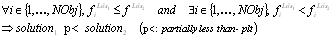(7-1)

If solution1 is p< (partially less than) solution2, it follows that solution1 dominates solution2. In the example used here this means: if costs and time are less for solution1 than for solution2, it follows that solution1 is superior to solution2. It would even be sufficient if one of the two values was equal for both solutions (equal costs) and only the other value was lower (less time).

If, however, none of the solutions dominates the other both solutions are to be regarded as equivalent with respect to the PARETO-order. The same rank is assigned to individuals which do not dominate each other.

The rank of an individual within the population (ranki) depends on the number of individuals (NumInddominated) dominating this individual [Fon95]: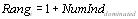(7-2)

All solutions that are found during optimization and are not dominated by a different solution constitute the PARETO-optimal solutions (PARETO-optimal set) of this problem (PARETO-optimality). These solutions are assigned a rank value of 1. In the case of each PARETO-optimal solution it is not possible to improve one of the criteria without one or several of the other criteria deteriorating.

## 7.2 Goal attainment or method of inequalities

When using plain PARETO-ranking in equation 7-1 all PARETO-optimal solutions are equivalent. It is possible, however, to make a further differentiation for many practical problems. As above, the example of object production shall be used to illustrate this. In an extreme case a solution could produce nothing at all. In this case, the costs would equal zero and the production time would be infinite. No other solution could produce the objects at lower costs. Thus, as this solution cannot be dominated, it would belong to the PARETO-optimal solutions if PARETO-dominance was applied exclusively. In a second extreme case a solution could produce objects in a very short time. This would result in very high expenditure, and therefore very high costs. It should be obvious that both cases are not desirable although they belong to the non-dominated solutions which are not dominated.

Goals for the individual criteria can be introduced in order to preclude PARETO-optimal set solutions, which lie outside of the results desired by the user. A solution is only acceptable when the goals for the individual criteria have been reached. This procedure is also termed method of inequalities , MOI, or goal programming. The individual goals are predefined as inequalities.

In the production example used here, this could mean that an upper limit for costs and production time is determined. The result is not acceptable until both goals are simultaneously reached or fallen short of by means of a solution.

When the goals are included in the multi-objective ranking the comparison of two solutions becomes somewhat more complex. The following assumptions are made: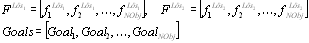(7-3)

In the definitions the operator partially less p< from equation 7-1 is used for each of the following definitions. It is possible to distinguish 3 different cases.

1. Solution1 does not fulfill any goals: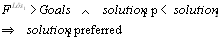(7-4)

2. Solution1 fulfills all goals: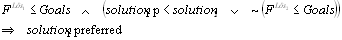(7-5)

3. Solution1 fulfills some goals: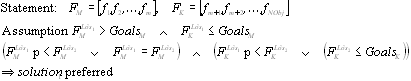(7-6)

PARETO-ranking using a vector of goals for the individual criteria, allows for an improved generation of the sequence of a number of solutions compared to plain PARETO-ranking.

## 7.3 Sharing in search space or in solution space

Multi-objective optimization is carried out in order to find a number of non-dominated solutions, the PARETO-optimal set. A normal evolutionary algorithm, however, converges at a single solution. This process is termed genetic drift. For this reason, methods must be built in, which achieve the maintenance or expansion of population diversity (prevention of premature convergence).

On the one hand, the genetic drift can be counteracted by the application of fitness sharing. On the other hand, the algorithm has the effect that a greater part of the pareto-optimal solution is ascertained. The basic principle is that individuals in a particular niche must share the resources available amongst themselves. In this way, the more individuals are near an individual, the lower its fitness becomes.

During application the size of the niches and the allocation of resources within each niche must be established. Methods for fitness sharing are suggested in [HN93], [SD94] and [Fon95], amongst others.

## 7.4 Further information on multi-objective optimization

In this subsection it was only possible to provide a short introduction to the multi-objective fitness assignment within the context of evolutionary algorithms. The relevant literature should be referred to for more specific questions regarding individual procedures: [Hor97], [Fon95], [ZT98], [HN93], [SD94], [Vel99]. A list of a large amount of literature on multi-objective optimization with evolutionary algorithms can be accessed in [Coe99]. There is a great deal of literature on multi-objective optimization not specific to the evolutionary algorithm. [Mie99] is recommended as a good starting point.

## 7.5 Weighted sum - aggregation or scalarization of multiple objectives

When considering different methods and component parts used for multi-objective optimization one should not forget classic methods for the integration of several criteria (scalarization method, also called aggregation of objectives).

The weighted sum is the most well-known method. Here each criterion is assigned a weighting value. By means of the linear combination of all the weighted criteria a combined objective function value Fws is achieved:(7-7)

The weighted sum is especially used in cases when the varying significance of the individual criteria is known or can be estimated. As this is frequently the case where practical application is concerned, the weighted sum is often applied.

If a multi-objective problem is solved by means of single-objective optimization, only a point solution is obtained. The advantage of obtaining several solutions of equal value relating to a target vector is lost. At this stage the user must decide whether the simple use of the weighted sum or the approximation of the PARETO-optimal solutions is more important for the solution of the problem.

 GEATbx: Main page  Tutorial  Algorithms  M-functions  Parameter/Options  Example functions  www.geatbx.com

This document is part of version 3.8 of the GEATbx: Genetic and Evolutionary Algorithm Toolbox for use with Matlab - www.geatbx.com.
The Genetic and Evolutionary Algorithm Toolbox is not public domain.